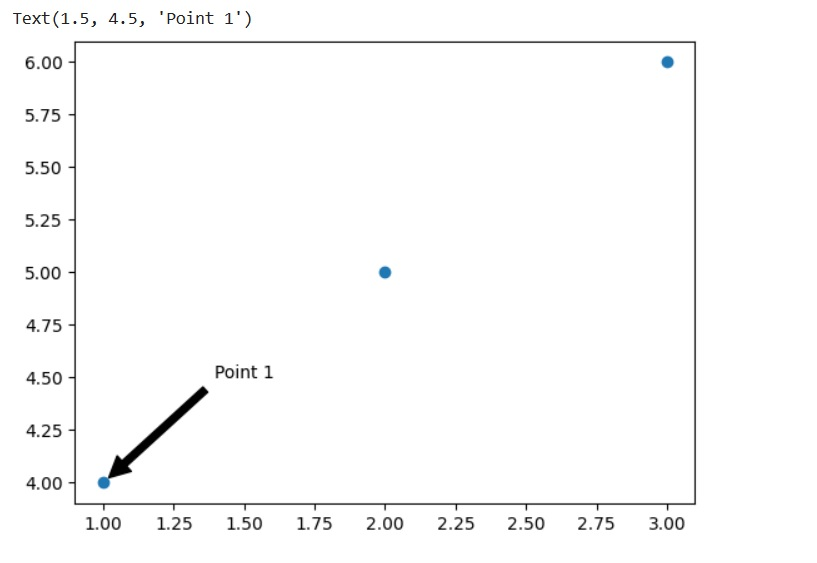### How to show point coordinates in a plot in Python using Matplotlib?

Matplotlib is a popular data visualization library in Python that allows you to create a wide range of charts and plots. To show point coordinates in a plot using Matplotlib, you can use the annotate() function, which allows you to add text annotations to your plot at specific coordinates.

The annotate() function takes several arguments, including the text to display, the xy position of the point, the text position in relation to the xy position, and the horizontal alignment of the text. By specifying the xy position of each point as the annotation position, you can display the point coordinates in your plot.

### Syntax

The syntax of the annotate() function in Matplotlib is as follows:

`annotate(s, xy, xytext=None, arrowprops=None, textcoords=None, ha='center')`

Here is an explanation of the arguments:

• s: A string that represents the text to display in the annotation.
• xy: A tuple that contains the (x, y) position of the point to annotate in data coordinates.
•  xytext: A tuple that contains the (x, y) position of the text in data or axes coordinates. If None, defaults to xy.
• arrowprops: A dictionary of properties that define the arrow. For example, you can specify the arrow color, style, width, and head length.
• textcoords: A string that specifies the coordinate system for xytext. The default is the same as xy.
• ha: A string that specifies the horizontal alignment of the text. The default is 'center'.

You can call the annotate() function on a Matplotlib axis object to add an annotation to your plot at the specified position.

For example:

`import matplotlib.pyplot as pltfig, ax = plt.subplots()ax.plot([1, 2, 3], [4, 5, 6], 'o')ax.annotate('Point 1', xy=(1, 4), xytext=(1.5, 4.5),                arrowprops=dict(facecolor='black', shrink=0.05),                    ha='center')`

#### OutputThis code will plot a scatter plot with three points, and add an annotation to the first point with the text "Point 1" and an arrow pointing to the text. The annotation will be positioned at (1.5, 4.5), which is an offset from the point (1, 4).

### Steps

Following are the steps to show point coordinates in a plot using Matplotlib in Python:

#### Step 1

Import the required libraries:
`import matplotlib.pyplot as plt`

#### Step 2

Create your data for the plot. This could be any set of x and y coordinates that you want to plot.

#### Step 3

Plot the data using the plot() function, and specify the marker style you want to use. For example, you could use the 'o' marker style to plot circles at each point.
`plt.plot(x, y, 'o')`

#### Step 4

Use a for loop to add an annotation for each point using the annotate() function. The function takes several arguments, including the text to display, the xy position of the point, the text position in relation to the xy position, and the horizontal alignment of the text. In the example below, we display the coordinates of each point as the annotation text.
```for i, j in zip(x, y):
plt.annotate('(%s, %s)' % (i, j), xy=(i, j), textcoords='offset points', xytext=(0,10), ha='center')```

#### Step 5

Show the plot using the show() function.
`plt.show()`

### Complete Program

`import matplotlib.pyplot as plt# create datax = [1, 2, 3, 4, 5]y = [2, 4, 6, 8, 10]# plot the dataplt.plot(x, y, 'o')# add annotations for each pointfor i, j in zip(x, y):    plt.annotate('(%s, %s)' % (i, j), xy=(i, j), textcoords='offset points', xytext=(0,10), ha='center')    # show the plotplt.show()`

#### Output

In this example, we first create some data for the x and y coordinates, and then plot the data using the plot function with 'o' as the marker style.

Then, we use a for loop to add an annotation for each point. The annotate function takes several arguments, including the text to display, the xy position of the point, the text position in relation to the xy position, and the horizontal alignment of the text.

Finally, we show the plot using the show function. When you run this code, you should see a plot with the point coordinates displayed above each point.